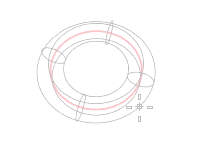# Torus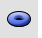Solid

Torus

The Torus command draws a torus (doughnut shape).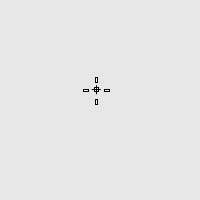Base circle options

Pick a point or enter a radius to decide the size of the base circle.### Vertical

Draws the base circle vertical to the construction plane.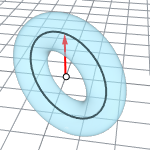### 2Point

Pick two opposite points on the base circle.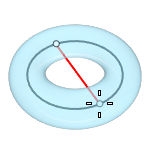### 3Point

Draws the base circle through three points.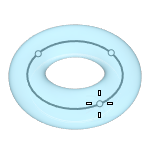### Tangent

Draws the base circle tangent to one, two, or three curves.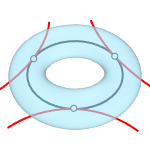### AroundCurve

Draws the base circle perpendicular to the picked point on a curve. The center line of the torus will be tangent to the curve.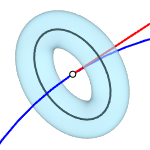### FitPoints

Draws the base circle by fitting to selected points, control points, or mesh vertices.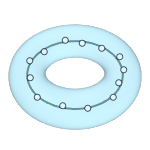Second circle options

Pick a point or enter a radius to decide the size of the second circle.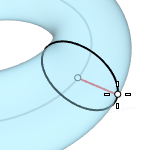### Diameter

Pick a point or enter a diameter to decide the size of the second circle.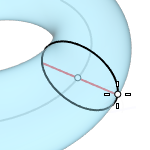### FixInnerDimension

The first radius chosen sets the inner dimension of the torus and the second radius is constrained to be outside of the first radius.